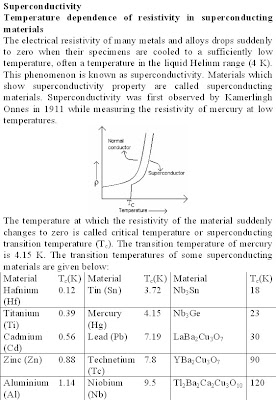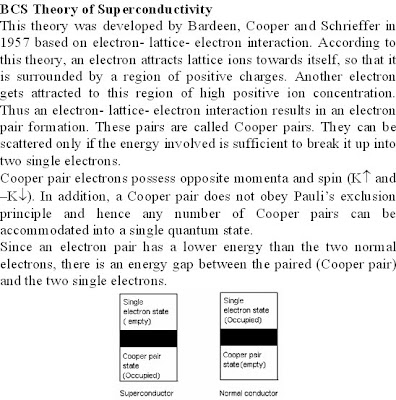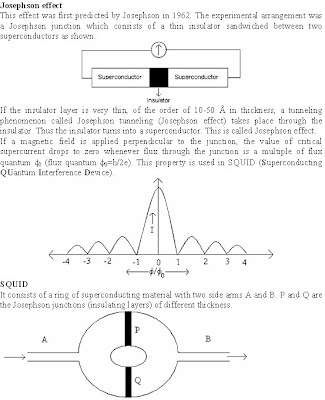Custom Search

## Friday, July 25, 2008

### Unit 6:Superconductivity and optical fibres

VTU Model Question paper

6.a.I Numerical aperture optical fiber depends upon

A) diameter of fiber B) acceptance angle C) critical angle D) none of these

II Fractional index change of optical fiber for R.I of core and cladding are 1.563 and 1.498 respectively is

A) 0.00415 B) 0.0415 C) 0.043 D) 0.004

III Superconductors are

A) diamagnetic B) paramagnetic C) ferromagnetic D) antiferromagnetic

IV The quantum magnetic flux is given by

A) 2h/e B) h/2e C) h/2p e D) 2p h/e

b. Derive an expression for numerical aperture in an optical fiber.

c. Give a brief account of SQUID.

d. An optical fiber has lost 85% of its power after traversing 500m in fiber. What is the loss in a fiber. (4+6+6+4)

December 08 / January 09

6 a. 1) The temperature at which superconductivity occurs is called

i) Low temperature ii) Super temperature iii) Critical temperature iv) High temperature.

2) Superconductivity phenomenon can be explained on the basis of

i) BCS theory ii) CCS theory iii) DCS theory iv) MCS theory.

3) Meissner effect will take place in

i) Solid ii) Super conducting magnet iii) Maglev vehicle iv) MRI.

4) Loss of power during transmission through optical fiber is called

i) Power loss ii) Energy loss iii) Attenuation iv) Modification. (04 Marks)

b. Explain Meissner effect. (06 Marks)

c. Obtain an expression for numerical aperture and arrive the condition for propagation. (06 Marks)

d. The angle of acceptance of an optical fiber is 30o when kept in air. Find the angle of acceptance when it is

in a medium of refractive index 1.33. (04 Marks)

June-July 2009

6 a. i) Numerical aperture of an optical fiber depends on

A) Diameter of the fiber B) Acceptance angle C) Critical angle D) hcore material

ii) The width of the energy gap of the superconductor is maximum at

A) Tc B) 0K C) Tc/2 D) Tc/3

iii) Which of the following is correct?

A) Cladding is for providing greater strength B) Core has higher R.I than cladding

C) Cladding has higher R.I than core D) None.

iv) Fractional index changes of optical fiber for R.I of core and cladding are 1.563 and 1.498 is

A) 0.00415 B) 0.0415 C) 0.043 D) 0.004 (04 Marks)

b. With neat figure derive an expression for N.A in an optical fiber. (06 Marks)

c. Give a brief account of SQUID (06 Marks)

d. A fiber 500m long has an input power of 8.6 mw and output power 7.5 mw. What is the loss specification

in cable? (04 Marks)

Dec09-Jan10

6 a. i) In a single mode fiber, the diameter of the core is nearly equal to

A) 125 mm B) 100 mm C) 50 mm D) 10 mm

ii) The numerical aperture of an optical fiber is 0.2 when surrounded by air. The acceptance angle when

the fiber is in water of refractive index 1.33 is

A) 8.21' B) 8.65' C) 0.11' D) None of these

iii) The loss of power by the optical signal through the optical fiber is mainly due to

A) Rayleigh scattering B) Raman scattering C) Wien’s scattering D) All of these

iv) When the type-I superconducting material is placed in an external magnetic field, it

A) attracts the magnetic lines B) enhances the magnetic flux lines

C) repels the magnetic flux lines D) does not influence magnetic field of lines (04 Marks)

b. Describe the point to point communication system, with the help of block diagram. (05 Marks)

c. Discuss BCS theory of superconductor. Explain SQUID. (07 Marks)

d. An optical glass fiber of refractive index 1.50 is to be clad with another glass to ensure internal reflection

that will contain light traveling within 5o of the fiber axis. What maximum index of refraction is allowed

May/June 2010

6 a. i) Superconductor in superconducting state behaves as

A) Monovalent metals B) Ferromagnetic materials

C) Good conductors at room temperature D) Diamagnetic materials

ii) A superconducting material, on being subjected to the critical field, changes to

A) Critical conductivity B) Superconducting which is independent of temp

C) Normal state D) Remains uninfluenced

iii) Fractional index change of optical fiber and refractive index of core are 0.00515 and 1.533

respectively. The cladding refractive index is

A) 1.492 B) 1.525 C) 1.499 D) 1.511

iv) Attenuation in the optical fiber causes due to

A) Absorption B) Scattering C) Dispersion D) All the three (04 Marks)

b. What is Meissner effect? Explain the BCS theory of superconductors. (08 Marks)

c. Derive the expression for numerical aperture of an optical fiber. (04 Marks)

d. An optical fiber has core R.I. 1.5 and R.I. of cladding 3% less than the core index. Calculate the numerical

aperture, angle of acceptance and internal critical acceptance angle. (04 Marks)

January 2011

6 a. i) The critical temperature of mercury is

A) 4.2 K B) 6.2 K C) 7.8 K D) 20 K

ii) The temperature of a superconductor kept in a weak magnetic field is reduced below critical

temperature, then

A) R=0;B≠0 B) R≠0;B=0 C) R≠0;B≠0 D) R=0;B=0

iii) The numerical aperture of an optical fiber in air is 0.32. The numerical aperture in water (n0 = 4/3) is

A) 0.43 B) 0.24 C) 0.64 D) 0.96

iv) Graded index fiber can be

A) single mode fiber only B) multimode fiber only

C) both single mode and multimode D) depends on the surrounding medium (04 Marks)

b. Define the terms: i) angle of acceptance ii) numerical aperture

iii) fractional index change iv) modes of propagation (04 Marks)

c. Explain BCS theory of superconductivity. Write a short note on maglev vehicles. (08 Marks)

d. The refractive indices of core and cladding are 1.50 and 1.48 respectively in an optical fiber. Find the

numerical aperture and angle of acceptance. (04 marks)

June/ July 2011

6 a. i) The conductivity of a superconductor is

A) infinite B) Zero C) finite D) none of these

ii) The relation between superconducting transition temperature (Tc) and atomic weight (m) of isotope is

A) Tc α m B) Tc α C) Tc α D) Tc α

iii) If optic fibre is kept in a medium of R.I m (>1) instead of air , the acceptance angle

A) increases B) decreases C) remains constant D) none of these

A) exponentially B) linearly C) parabolically D) none of these

(04 Marks)

b. Discuss types of optical fibres and modes of propagation using suitable diagram. (06 Marks)

c. Distinguish between type-1 and type-11 super conductors. (05 Marks)

d. The angle of acceptance of an optical fibre is 300 when kept in air. Find the angle of acceptance when it

is medium of R.I. 1.33. (05 Marks)

For objective type questions http://vtuphysicsobjective.blogspot.com/2009/03/unit6.htmlsicsobjective.blogspot.com/2009/03/unit6.htmlAs long as Cooper pair electrons remain in Cooper pair states, they do not suffer scattering and hence resistivity will be zero. When the temperature is raised, to overcome the energy gap, Cooper pair electrons gets separated to normal single electrons which may undergo scattering due to the presence of imperfections in the crystal or lattice vibrations, which leads to a finite resistivity.
High temperature superconductors
In 1986, Bednorz and Muller synthesized a particular type of ceramic material (LaBa2Cu3O7) whose transition temperature was 30 K. For this they were awarded the Nobel Prize in Physics in the year 1987. Later researchers synthesized YBa2Cu3O7 with Tc around 90 K. The success broke the barrier of liquid nitrogen temperature of 77 K, and was a sort of dream come true for many scientists. It is because liquid nitrogen is readily available in most of the places and inexpensive.
All high temperature superconductors are different types of oxides of copper assuming perovskite crystal structure. The critical temperature is higher for those materials which have more number of copper-oxygen layers. The formation of supercurrents in high temperature superconductors is direction dependent. The supercurrents are strong in the copper-oxygen planes and weak in a direction perpendicular to the planes. In bulk materials, the grain boundary effects decreases the critical current value.
Applications of superconductorsLet dp and dq represent the phase difference between the input current and output current while passing through the insulator junctions P and Q respectively. In the absence of magnetic field, these two phases are equal. i.e. dp = dq = d0.
When a magnetic field is applied, the phase difference between the reunited currents is directly proportional to the magnetic flux f passing through the ring. It can be shown that total current coming out of the ring,
I = 2I0 sind0 cos(ef/hc)
This expression indicates that the output current varies with the applied magnetic flux and shows oscillations.
SOUID is used as a very sensitive magnetometer which can measure very weak magnetic fields of the order of 10-13 Tesla.
Other applications of superconductors
1.In high energy physics experiments.
2.In NMR imaging.
3.In magneto hydrodynamic power generation.
4.In magnetic separation for refining ores and chemicals.
5.memory storage element in computers.
6.In superconducting generators and motors.
7.In superconducting fuses, switches and cables.Types of optical fibres

Different mechanisms of attenuation
1. Absorption: The optical fibre material and the impurities present in the material absorb light leading to fibre loss.
2. Rayleigh scattering: This occurs due to the local variations in refractive index. The Rayleigh scattering loss depends on the wavelength. It varies as 1/l4 and becomes significant at lower wavelengths. Below 0.8mm, the scattering loss is very high.
3. Radiation losses: This occurs due to the bending of the fibre. There are two types of bends-Macroscopic and Microscopic.
Dispersion losses due to various modes of propagation
1) Waveguide dispersion
In single mode fibres, part of the light ray will be refracted into the cladding. The loss due to this is referred as waveguide dispersion. Waveguide dispersion is negligible in multimode fibres.
2) Intermodal dispersion
The intermodal dispersion occurs in multimode fibres where rays associated with various modes travel different distances through the fibre. As a result, the signal broadens and the output signal is no longer identical with the input signal. Signal broadening is less in graded index and step index single mode fibres.
3) Material dispersion or chromatic dispersion
If we use white light, all the colours of the input radiation are not reaching the other end at the same time since they travel with different velocities. Signal distortion of this kind is called material dispersion or chromatic dispersion.
Applications of optical fibres
1. In fibre optic communication
2. In fibre optic sensors
3. For industrial automation
4. In security alarm systems
5. In local area network (LAN) of computers
6. For high speed data transmission in computers
7. Medical applications- for diagnosis and surgical applications (endoscopy)
8. Military applications (fibre guided missiles)
Point to point light wave communication using optic fibre
A simple block diagram of fibre optic communication system is shown below:

Optical transmitter
A light emitting diode (LED) or a semiconductor laser can be used as optical source. Modulation modulates the input signal and optical signal and then transmitted through optical fibre cables to the receiver.
A photodiode can be used as optical detector. The detected wave is demodulated to extract the signal.
1) Wide band width.
2) Low attenuation and other transmission losses.
3) Small size and weight.
4) Safe from electrical interference caused by lightning, electric motors, fluorescent tube and other electrical noise sources.
5) Lack of cross talk between parallel fibres.
6) Easy installation and easy maintenance.
7) Flexible.
8) Temperature resistance.
9) Highly economical.
10) High degree of signal security.
11) Longer life span.